• 如果您觉得本站非常有看点，那么赶紧使用Ctrl+D 收藏吧

# 【小白学PyTorch】11 MobileNet详解及PyTorch实现

6天前 16次浏览

@

• 1 背景
• 2 深度可分离卷积
• 2.2 一般卷积计算量
• 2.2 深度可分离卷积计算量
• 2.3 网络结构
• 3 PyTorch实现

## 2 深度可分离卷积

• 深度卷积(depthwise convolution)
• 逐点卷积(pointwise convolution)。
这么做的好处是可以大幅度降低参数量和计算量。

### 2.2 一般卷积计算量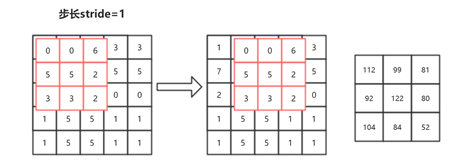(9*9=3*3*3*3=81)

(9*9*2=3*3*3*3*2=162)

(9*9*2*3=3*3*3*3*2*3=486)

(H*W*K*K*M*N)

### 2.2 深度可分离卷积计算量

• 深度可分离卷积（Depthwise Separable Convolution，DSC）

(7*7*3*3*64*128=3612672)

1. Depthwise：先用64×7×7经过3×3的卷积核得到一个64×7×7的特征图。注意注意！这里是64×7×7的特征图经过3×3的卷积核，不是64×3×3的卷积核！这里将64×7×7的特征图看成64张7×7的图片，然后依次与3×3的卷积核进行卷积；
2. Pointwise：在Depthwise的操作中，不难发现，这样的计算根本无法整合不同通道的信息，因为上一步把所有通道都拆开了，所以在这一步要用64×1×1的卷积核去整合不同通道上的信息，用128个64×1×1的卷积核，产生128×7×7的特征图。

(7*7*3*3*64+7*7*1*1*64*128=429632)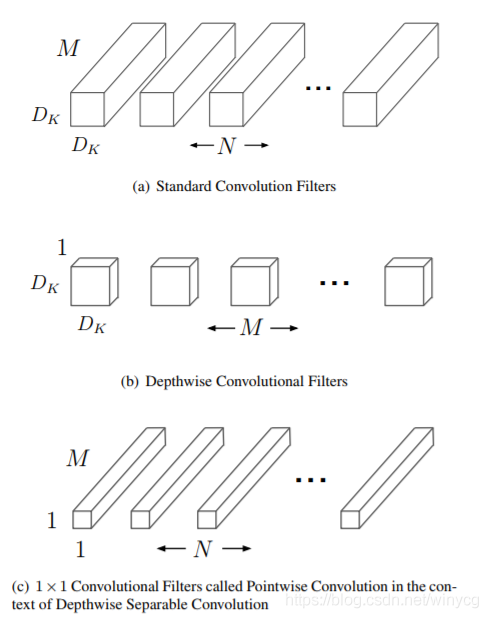• (a)表示一般卷积过程， 卷积核都是M个通道，然后总共有N和卷积核，意味着输入特征图有M个通道，然后输出特征图有N个通道。
• (b)表示depthwise过程， 总共有M个卷积核，这里是对输入特征图的M个通道分别做一个卷积，输出的特征图也是M个通道的；
• (c)表示pointwise过程，总共有N个(1 times 1)的卷积核，这样来整合不同通道的信息，输出特征图有N个通道数。

### 2.3 网络结构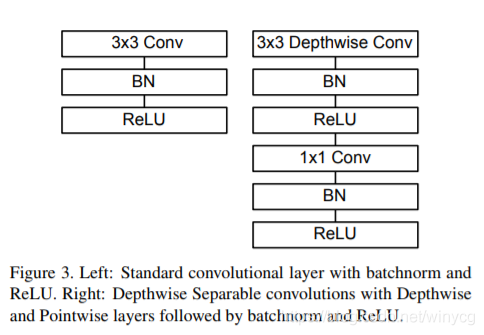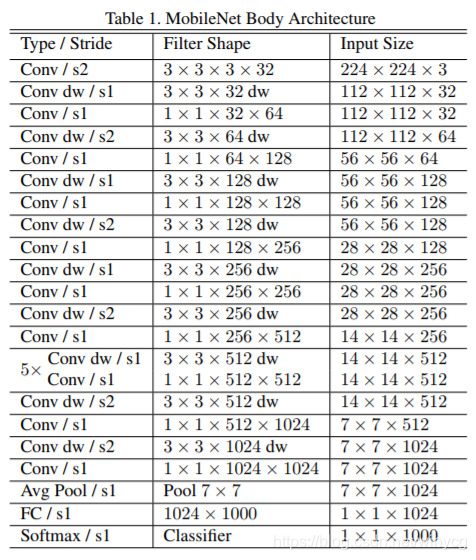• 除了第一层为标准的卷积层之外，其他的层都为深度可分离卷积。
• 整个网络没有使用Pooling层。

## 3 PyTorch实现

``````import torch
import torch.nn as nn
import torch.nn.functional as F

class Block(nn.Module):
'''Depthwise conv + Pointwise conv'''
def __init__(self, in_planes, out_planes, stride=1):
super(Block, self).__init__()
self.conv1 = nn.Conv2d
(in_planes, in_planes, kernel_size=3, stride=stride,
self.bn1 = nn.BatchNorm2d(in_planes)
self.conv2 = nn.Conv2d
(in_planes, out_planes, kernel_size=1,
self.bn2 = nn.BatchNorm2d(out_planes)

def forward(self, x):
out = F.relu(self.bn1(self.conv1(x)))
out = F.relu(self.bn2(self.conv2(out)))
return out

class MobileNet(nn.Module):
# (128,2) means conv planes=128, conv stride=2,
# by default conv stride=1
cfg = [64, (128,2), 128, (256,2), 256, (512,2),
512, 512, 512, 512, 512, (1024,2), 1024]

def __init__(self, num_classes=10):
super(MobileNet, self).__init__()
self.conv1 = nn.Conv2d(3, 32, kernel_size=3,
self.bn1 = nn.BatchNorm2d(32)
self.layers = self._make_layers(in_planes=32)
self.linear = nn.Linear(1024, num_classes)

def _make_layers(self, in_planes):
layers = []
for x in self.cfg:
out_planes = x if isinstance(x, int) else x
stride = 1 if isinstance(x, int) else x
layers.append(Block(in_planes, out_planes, stride))
in_planes = out_planes
return nn.Sequential(*layers)

def forward(self, x):
out = F.relu(self.bn1(self.conv1(x)))
out = self.layers(out)
out = F.avg_pool2d(out, 2)
out = out.view(out.size(0), -1)
out = self.linear(out)
return out

net = MobileNet()
x = torch.randn(1,3,32,32)
y = net(x)
print(y.size())
> torch.Size([1, 10])
``````

``````print(net)
``````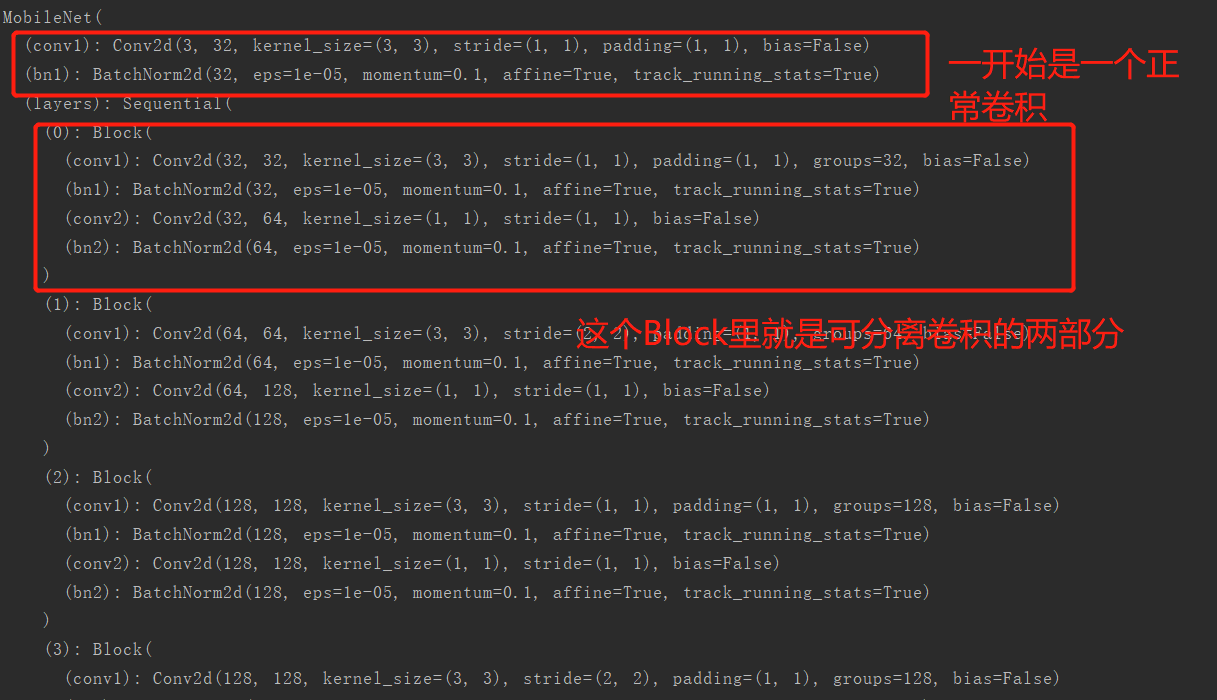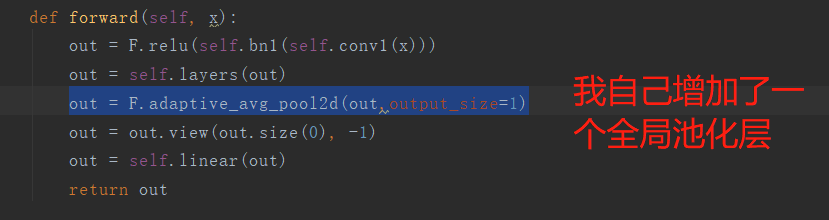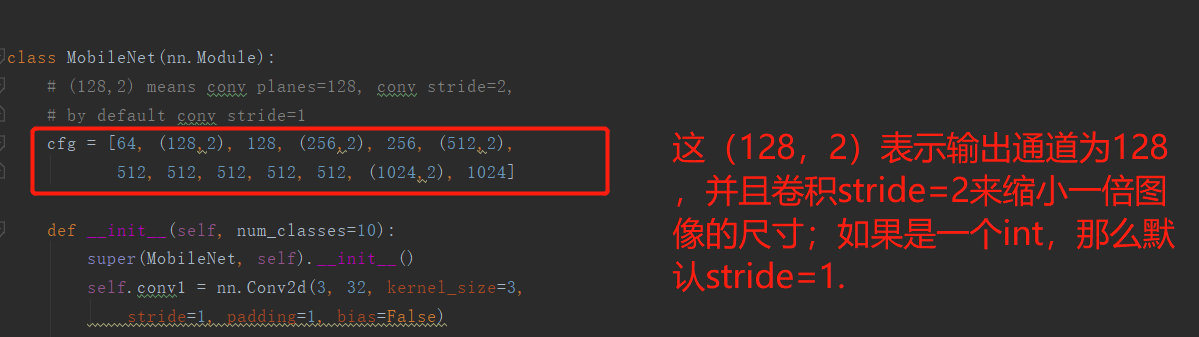MobileNet就差不多完事了，下一节课为SENet的PyTorch实现和详解。